# Space Structure on Elastic SupportThis test lets you check space structure on elastic support, in the context of a static case. You will use 1D mesh. This test is used to validate the discrete elastic coupling.Reference:

AFNOR technique, SFM Paris, Guide de validation des progiciels de calcul de structures, SSLL 04/89, pp.26-27, 1990.

##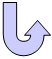Specifications

### Geometry Specifications

 Length: L = 2000 mm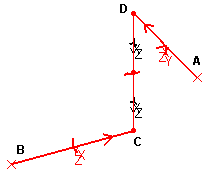Inertia: Ix/2 = Iy = Iz = 10-6 m4 Area: a = 10-3 m2

### Analysis Specifications

 Young Modulus (material): E = 210 GPa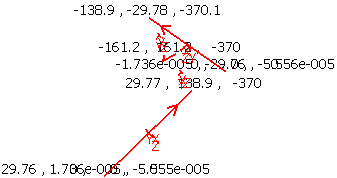Poisson's Ratio (material): ν= 0.333333 Loads (traction at D): Distributed force Fz = -10000 N Restraints: Displacement Type: at A: four springs (one in translation, three in rotation, one of which is infinitely rigid)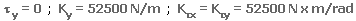at B: four springs (one in translation, three in rotation, one of which is infinitely rigid)at H: ball joint (articulation with all three rotations released) Traction Type: at D: Fz = -10000 N

##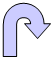Results

The results correspond to the quantity at each point and according to x, y, or z.
The table below presents the beam output results.

 Point Quantity Reference Computed result A Mx [N_m] -8437.5 -8438.14 A My [N_m] -1562.5 -1562.3 A Mz [N_m] 3125.0 3124.6 B Mx [N_m] 1562.5 1562.5 B My [N_m] 8437.5 8437.05 B Mz [N_m] 3125.0 3125.0 A v [mm] -29.76 -29.76 A θ [deg] 9.208 9.209 D w [mm] 370.04 370.07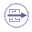To Perform the Test:

The SpaceStructure.CATAnalysis document presents a complete analysis of this case, computed with a mesh formed of beam elements. Proceed as follow:

1. Open the CATAnalysis document.

2. Compute the case and generate an image called Deformed mesh.